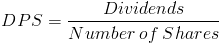# Dividends Per ShareThe formula for dividends per share, or DPS, is the annual dividends paid divided by the number of shares outstanding.

## Per Share

The denominator of the dividends per share formula generally uses the annual weighted average of outstanding shares. The weighted average is also used with the earnings per share formula. However, there are key differences between these two formulas. The numerator for earnings per share is net income, or earnings. The numerator for the dividends per share formula is dividends. Earnings is effectively a continuous process throughout the year whereas dividends are paid at a given moment.

How often a company pays a dividend may warrant consideration for how to calculate the per share portion of the formula when using financial analysis for investments.

An unlikely figurative example would be a company who paid dividends in January with 2,000 outstanding shares and issued 20,000 additional shares in December. The result of the dividends per share formula would vary greatly depending on which method is used for determining the number of shares outstanding. Considering that the dividend yield formula uses dividends per share, it would vary greatly as well.

However, another hypothetical company pays dividends monthly and has issued common shares periodically throughout the year. One may consider using the weighted average in this example.

As with most financial formulas, perspective is important.

## Use of DPS Formula

Dividends per share and the formula provided may be used by individuals who are evaluating various stocks to invest in and prefer companies who pay dividends. This formula alone does not necessarily provide an overall outlook on a company as some companies retain their earnings for growth instead of paying dividends. A company with a low dividend payout ratio, i.e. a company who pays a smaller percentage of their net income to stockholders, will reinvest their net income which may lead to an increase in the value of the company due to expansion.

Dividends per share is also used in other financial formulas, including dividend yield and dividend payout ratio.

New to Finance?